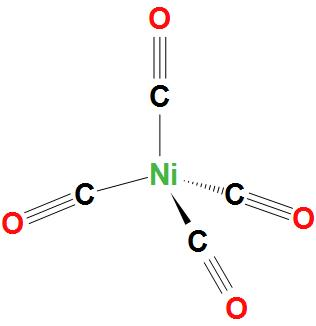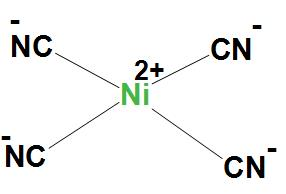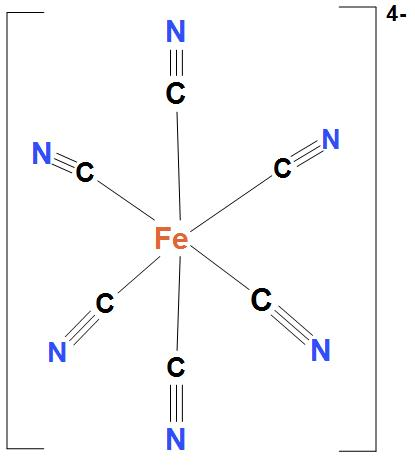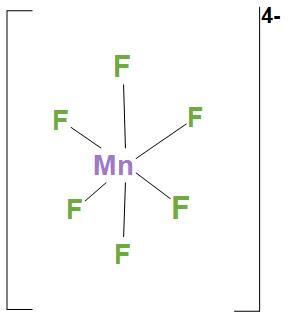# Match list-I (complexes) with list-II (Hybridization of central atom) and select the correct answer using the codes given below the lists: List-I List-IIA. $Ni{{\left( CO \right)}_{4}}$ 1. $s{{p}^{3}}$B. $Ni\left( CN \right)_{4}^{2-}$ 2. $ds{{p}^{2}}$C. $Fe\left( CN \right)_{6}^{4-}$ 3. $s{{p}^{3}}{{d}^{2}}$D. $MnF_{6}^{4-}$ 4. ${{d}^{2}}s{{p}^{3}}$5. $s{{p}^{2}}d$...A. A-1, B-3, C-2, D-4B. A-5, B-2, C-4, D-3C. A-5, B-3, C-2, D-4D. A-1, B-2, C-4, D-3Verified
183.3k+ views
Hint As we know that hybridization is the process of producing a degenerated type of orbital by mixing two atomic orbitals with the same energy levels. During hybridization, atomic orbitals are mixed and a new hybrid orbital is formed.

Complete Step by step solution:
- In $Ni{{\left( CO \right)}_{4}}$, Ni atom is $s{{p}^{3}}$ hybridised. And this will lead to a tetrahedral geometry. We can see the structure:- In $Ni\left( CN \right)_{4}^{2-}$, Ni atom is $ds{{p}^{2}}$ hybridised. And this will lead to a square planar geometry.
Here, $Ni\left( CN \right)_{4}^{2-}$ is showing $ds{{p}^{2}}$ hybridisation, because here CN group is a strong field ligand and it forms inner complexes. We can see the structure:- In $Fe\left( CN \right)_{6}^{4-}$, Ni atom is ${{d}^{2}}s{{p}^{3}}$ hybridised. And this will lead to an octahedral geometry. Here, $Fe\left( CN \right)_{6}^{4-}$ is showing ${{d}^{2}}s{{p}^{3}}$ hybridisation, because here cyanide group is present. And as we know that cyanide group is a strong field ligand and it forms inner complexes. We can see the structure:- In $MnF_{6}^{4-}$, Ni atom is $s{{p}^{3}}{{d}^{2}}$ hybridised. And this will lead to an octahedral geometry. We can see the structure:Hence, we can say that correct option is (d), that is A-1, B-2, C-4, D-3

Note:
- We must note the key features of hybridization: the atomic orbitals that have equal energies undergo hybridization. Shape of any molecule can be predicted from hybridization.
- The number of hybrid orbitals that are formed during hybridization will be equal to the number of atomic orbitals mixed.